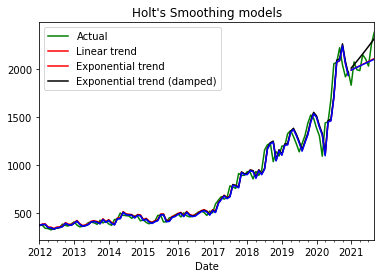# Reliance stock price forcasting 2023

## How to get reliance data from Yahoo Finance in Python?

Forecasting the stock price of Reliance Industries Limited (Reliance) or any other stock involves predicting its future price movements based on historical data, technical analysis, fundamental analysis, and sometimes machine learning models. Forecasting the stock price of Reliance Industries Limited, like any other publicly traded company, involves a combination of fundamental and technical analysis, market sentiment, and external factors.
Here's a general overview of how one might approach forecasting the stock price of Reliance:

Fundamental Analysis: This involves examining the financial health and performance of Reliance Industries. Key factors include:

a. Earnings Reports: Review quarterly and annual financial reports to assess revenue growth, profit margins, and earnings per share (EPS).

b. Balance Sheet: Analyzing the company's assets, liabilities, and equity to gauge its financial stability and leverage.

c. Cash Flow: Evaluating the company's cash flow statements to understand its ability to generate cash and fund operations and investments.

d. Dividends: Consideration of dividend history and the company's dividend policy, which can impact investor sentiment.

e. Competitive Position: Assessing how Reliance Industries compares to competitors in its industry, including market share, product diversification, and growth prospects.

f. Industry Trends: Understanding trends and dynamics in the industries in which Reliance operates, such as telecommunications, petrochemicals, and retail.

Technical Analysis: Technical analysts use historical stock price charts, patterns, and technical indicators to predict future price movements. Common tools include moving averages, Relative Strength Index (RSI), and Bollinger Bands.

Market Sentiment: Sentiment analysis involves assessing how investors perceive Reliance Industries and its stock. News, social media, and analyst reports can provide insight into market sentiment.

External Factors: Factors beyond Reliance's control can influence its stock price. These include changes in government regulations, economic conditions, global commodity prices (since Reliance has interests in petrochemicals), and geopolitical events.

Management and Strategy: Evaluating the leadership of Reliance Industries, including the vision and strategies of its top executives, can provide insights into the company's future prospects.

Macroeconomic Trends: Broader economic indicators, such as GDP growth, inflation rates, and interest rates, can affect the overall stock market and, by extension, Reliance's stock.

Analyst Forecasts: Analysts from financial institutions often provide forecasts and recommendations for Reliance Industries' stock. These can be valuable inputs into the forecasting process.

Machine Learning and AI: Some investors and analysts use advanced techniques, including machine learning and artificial intelligence, to model and predict stock price movements based on historical data and various factors.

It's essential to remember that stock price forecasting is inherently uncertain, and past performance is not necessarily indicative of future results. Therefore, investors should use forecasts as one of many tools to inform their investment decisions and consider diversifying their portfolios to manage risk.

Additionally, consulting with financial professionals, conducting thorough research, and staying informed about the latest developments related to Reliance Industries and its industry can help in making more informed investment decisions.
Here are steps you can follow to create a basic stock price forecast for Reliance in Python:

Import Libraries:
Start by importing the necessary Python libraries for data analysis, visualization, and modeling:

Python
Copy code
import pandas as pd
import numpy as np
import matplotlib.pyplot as plt
import yfinance as yf
from sklearn.model_selection import train_test_split
from sklearn.linear_model import LinearRegression
Data Retrieval:
Use the yfinance library or another financial data source to fetch historical price data for Reliance:

Python
Copy code
Data Preprocessing:
Preprocess the data by selecting the relevant features (usually 'Adj Close' price), and create a target variable by shifting the price to represent future values. You can also add additional features if needed.

Python
Copy code
reliance['Next_Close'] = reliance['Adj Close'].shift(-1)  # Shift the price for prediction
Data Visualization:
Visualize the historical price data to get an idea of Reliance's price trends:

Python
Copy code
plt.figure(figsize=(12, 6))
plt.plot(reliance.index, reliance['Adj Close'], label='Reliance Stock Price')
plt.title('Reliance Stock Price')
plt.xlabel('Date')
plt.ylabel('Price')
plt.legend()
plt.show()
Feature Selection:
Select the features you want to use for forecasting. In this basic example, you can use 'Adj Close' as the only feature.

Data Splitting:
Split the dataset into training and testing sets. The training set will be used to train the model, and the testing set will be used to evaluate its performance:

Python
Copy code
y = reliance['Next_Close']
X_train, X_test, y_train, y_test = train_test_split(X, y, test_size=0.2, random_state=42)
Model Building:
Build a simple linear regression model to forecast future prices based on historical prices:

Python
Copy code
model = LinearRegression()
model.fit(X_train, y_train)
Model Evaluation:
Evaluate the model's performance on the testing data:

Python
Copy code
y_pred = model.predict(X_test)
Visualization of Predictions:
Visualize the model's predictions compared to the actual prices:

Python
Copy code
plt.figure(figsize=(12, 6))
plt.plot(reliance.index[-len(y_test):], y_test, label='Actual Prices', color='blue')
plt.plot(reliance.index[-len(y_test):], y_pred, label='Predicted Prices', color='red')
plt.title('Reliance Stock Price Prediction')
plt.xlabel('Date')
plt.ylabel('Price')
plt.legend()
plt.show()
Forecasting:
Use the trained model to make forecasts for future prices based on new data. You can predict the next day's price or multiple days into the future.

Please note that this is a basic example of stock price forecasting using linear regression. More complex models and additional features may be required for accurate forecasting. Additionally, stock price forecasting is inherently uncertain, and predictions should be used cautiously for investment decisions.
progress =False)

### There are a number of alternative sources like Quandal, Intinio, and google.

df.reset_index(drop=False, inplace=True)
df=DataFrame(df)
df
indexdsOpenHighLowCloseyVolume
002012-01-02345.128540351.542725340.348846349.957764323.0367748679938
112012-01-03352.780975360.037201351.839905358.922760331.3121039455771
222012-01-04360.284851362.043182353.325836354.712677327.4259348557084
332012-01-05354.143066359.071350343.791199346.465851319.81347713364666
442012-01-06345.252350358.600830345.054230355.406097328.0659489495456
...........................
237723772021-08-302250.0000002275.8500982236.8000492270.2500002270.2500006473487
237823782021-08-312276.8999022283.7500002242.2500002258.1499022258.14990212223037
237923792021-09-012273.0000002292.8999022263.0000002267.1000982267.1000985143640
238023802021-09-022255.0000002307.8000492255.0000002294.3999022294.3999024595048
238123812021-09-032310.0000002395.0000002302.5000002388.5000002388.50000014151629

2382 rows × 8 columns

train_indices = df.ds.apply(lambda x: x.year) < 2021
df_train = df.loc[train_indices].dropna()
df_test = df.loc[~train_indices].reset_index(drop=True)
fourier_order=5)

### How to plot the close price of 2022 reliance?

model_prophet.fit(df_train)
df_future = model_prophet.make_future_dataframe(periods=365)
df_pred = model_prophet.predict(df_future)
model_prophet.plot(df_pred)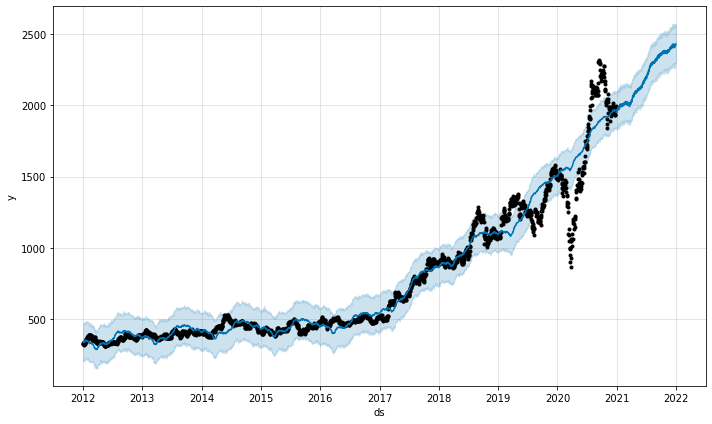model_prophet.plot_components(df_pred)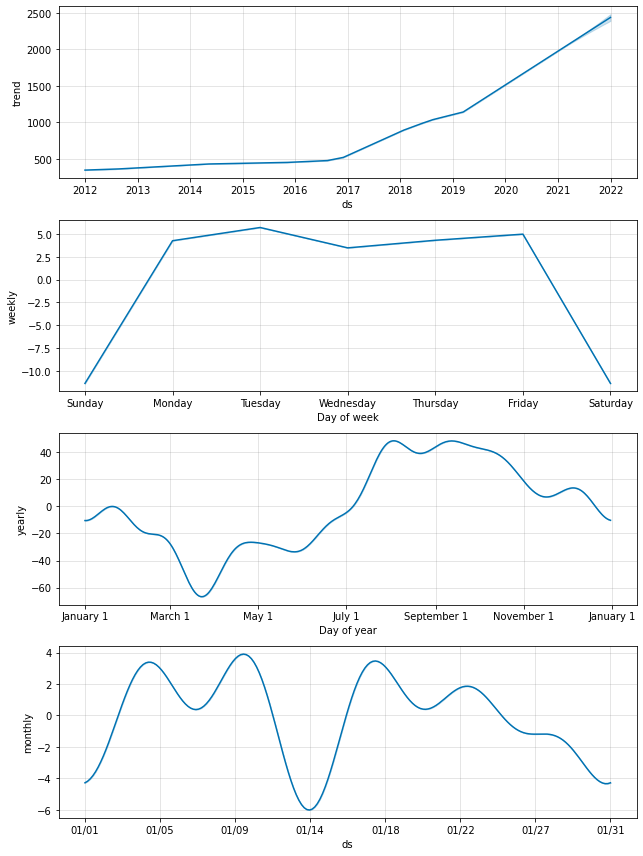progress =False)
import pandas as pd
import numpy as np
from pandas import Series, DataFrame
data=DataFrame(df_yah)
data

Date
2012-01-02345.128540351.542725340.348846349.957764323.0367748679938
2012-01-03352.780975360.037201351.839905358.922760331.3121039455771
2012-01-04360.284851362.043182353.325836354.712677327.4259348557084
2012-01-05354.143066359.071350343.791199346.465851319.81347713364666
2012-01-06345.252350358.600830345.054230355.406097328.0659489495456
.....................
2021-08-302250.0000002275.8500982236.8000492270.2500002270.2500006473487
2021-08-312276.8999022283.7500002242.2500002258.1499022258.14990212223037
2021-09-012273.0000002292.8999022263.0000002267.1000982267.1000985143640
2021-09-022255.0000002307.8000492255.0000002294.3999022294.3999024595048
2021-09-032310.0000002395.0000002302.5000002388.5000002388.50000014151629

2382 rows × 6 columns

DataFrame(data, columns=['Close''Volume'])

CloseVolume
Date
2012-01-02349.9577648679938
2012-01-03358.9227609455771
2012-01-04354.7126778557084
2012-01-05346.46585113364666
2012-01-06355.4060979495456
.........
2021-08-302270.2500006473487
2021-08-312258.14990212223037
2021-09-012267.1000985143640
2021-09-022294.3999024595048
2021-09-032388.50000014151629

2382 rows × 2 columns

frame2=DataFrame(data,columns=['Close'])
frame2

Close
Date
2012-01-02349.957764
2012-01-03358.922760
2012-01-04354.712677
2012-01-05346.465851
2012-01-06355.406097
......
2021-08-302270.250000
2021-08-312258.149902
2021-09-012267.100098
2021-09-022294.399902
2021-09-032388.500000

2382 rows × 1 column

from plotly.offline import iplot, init_notebook_mode
init_notebook_mode()
goog = data.resample('M') \
.last() \
goog  # convert daily into monthly goog(RIL)
Date 2012-01-31 373.580566 2012-02-29 375.249329 2012-03-31 343.176544 2012-04-30 340.661957 2012-05-31 326.532379 ... 2021-05-31 2153.372803 2021-06-30 2110.649902 2021-07-31 2035.300049 2021-08-31 2258.149902 2021-09-30 2388.500000 Freq: M, Name: adj_close, Length: 117, dtype: float64
train_indices = goog.index.year < 2021
goog_train = goog[train_indices]
goog_test = goog[~train_indices]
test_length = len(goog_test)
goog.plot(title="Relian's Stock Price");

### How to plot daily stock returns?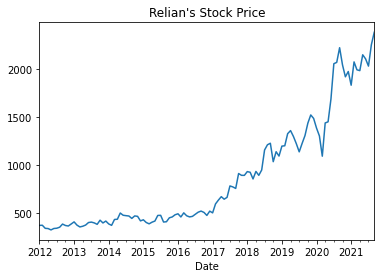ses_1 = SimpleExpSmoothing(goog_train).fit(smoothing_level=0.2)
ses_forecast_1 = ses_1.forecast(test_length)
ses_2 = SimpleExpSmoothing(goog_train).fit(smoothing_level=0.5)
ses_forecast_2 = ses_2.forecast(test_length)
ses_3 = SimpleExpSmoothing(goog_train).fit()
alpha = ses_3.model.params['smoothing_level']
ses_forecast_3 = ses_3.forecast(test_length)

goog.plot(color="g",
title='Simple Exponential Smoothing',
label='Actual',
legend=True)
ses_forecast_1.plot(color="r", legend=True,
label=r'$\alpha=0.2$')
ses_1.fittedvalues.plot(color="r")
ses_forecast_2.plot(color="k", legend=True,
label=r'$\alpha=0.5$')
ses_2.fittedvalues.plot(color="k")
ses_forecast_3.plot(color="b", legend=True,
label=r'$\alpha={0:.4f}$'.format(alpha))
ses_3.fittedvalues.plot(color="b")

### How to plot simple exponential smoothing daily stock return?

<matplotlib.axes._subplots.AxesSubplot at 0x7f9fc58b0150>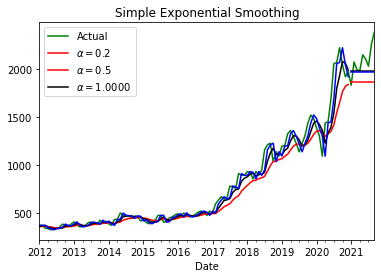Fit three variants of Holt's smoothing model and create forecasts:

# Holt's model with linear trend

hs_1 = Holt(goog_train).fit()
hs_forecast_1 = hs_1.forecast(test_length)
hs_2 = Holt(goog_train, exponential=True).fit()  # Holt's model with exponential trend
hs_forecast_2 = hs_2.forecast(test_length)
hs_3 = Holt(goog_train, exponential=False,      # Holt's model with exponential trend and damping
damped=True).fit(damping_slope=0.99)
hs_forecast_3 = hs_3.forecast(test_length)
goog.plot(color="g",
title="Holt's Smoothing models",
label='Actual',
legend=True)
hs_1.fittedvalues.plot(color="r")
hs_forecast_1.plot(color="r", legend=True,
label='Linear trend')
hs_2.fittedvalues.plot(color="k")
hs_forecast_2.plot(color="k", legend=True,
label='Exponential trend')
hs_3.fittedvalues.plot(color="b")
hs_forecast_3.plot(color="b", legend=True,
label='Exponential trend (damped)')

### how to plot reliance on Holt's smoothing model?

<matplotlib.axes._subplots.AxesSubplot at 0x7f9fc6231dd0>